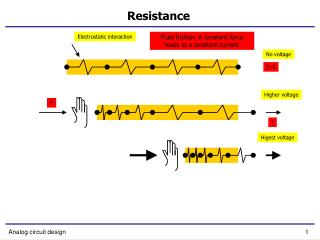DownloadDownload PresentationResistance

# Resistance

Télécharger la présentation## Resistance

- - - - - - - - - - - - - - - - - - - - - - - - - - - E N D - - - - - - - - - - - - - - - - - - - - - - - - - - -
##### Presentation Transcript

1. Resistance Electrostatic interaction Fluid friction. A constant force leads to a constant current No voltage I=0 Higher voltage F I Higest voltage Analog circuit design

2. Resistance (2) No voltage I=0 Voltage F I U Analog circuit design

3. Capacitor + + I -I U U An „analogy“ to capacitor Smaller voltage Higher voltage Analog circuit design

4. Kg Kg Kg Kg Kg Kg Kg Kg Kg Kg Kg Kg Inductance Intertia instead friction In the case of constant force, the current inctreases constantly No voltage I=0 Voltage F I ≈ 0, t = 0 I>>0, Später Analog circuit design

5. Amplifier Power supply Vout Resistor Operating point Operating point Vin Analog circuit design

6. Amplifier Vout Higher potential Lower potential than DC Vin Analog circuit design

7. Amplifier - linearity Vout Vin Analog circuit design

8. Amplifier - linearity Vout Vin Analog circuit design

9. Amplifier - linearity Vout Vin Analog circuit design

10. Amplifier - linearity Vout Vin Analog circuit design

11. Amplifier - linearity Vout Vin Analog circuit design

12. Amplifier - linearity Vout Vin Analog circuit design

13. Amplifier - linearity Vout Vin Analog circuit design

14. Amplifier – small signal gain Vout Slope of the line is small signal gain Vout Vin Vin Analog circuit design

15. Amplifier – dynamic range Vout Output dynamic range Input dynamic range Vin Analog circuit design

16. Amplifier – fine balanced amplifier Vout Antenna Reference point Vin Analog circuit design

17. Amplifier – unbalanced amplifier Vout Vin Analog circuit design

18. Amplifier – unbalanced amplifier Vout Vin Analog circuit design

19. Amplifier – stabilizing of operating point Vout Input of voltage divider Voltage divider Vin Output of voltage divider Analog circuit design

20. Amplifier – dimensioning of volt. div Vout Vin Analog circuit design

21. Amplifier – dimensioning of volt. div Vout Vin Analog circuit design

22. Amplifier – stabilizing of operating point Vout Vin Analog circuit design

23. Amplifier – stabilizing of operating point Vout Vin Analog circuit design

24. Amplifier – stabilizing of operating point Vout Vin Analog circuit design

25. Amplifier – stabilizing of operating point Vout Vin Analog circuit design

26. Amplifier – gain with feedback Vout Vin Analog circuit design

27. Amplifier – gain with feedback Vout DC point Vin Signal Analog circuit design

28. Amplifier – gain with feedback Vout Vin Analog circuit design

29. Amplifier – gain with feedback Vout Vin Analog circuit design

30. Amplifier – gain with feedback Vout Output signal Signal DC Vin Analog circuit design

31. Feedback analysis Xo Passive network Xi Xi* Passive network Xs Amplifier Input signal source Output Feedback The cut Analog circuit design

32. Test circuits Measurement-point - blue Short Test-source - red AIN – Gain in input network A – active Gain  - Feedback FF – feed forward T – loop gain Analog circuit design

33. Output impedance Current is measured Passive network Xi Xi* Passive network Xo=Io Xs=Vs Voltage sorce at output Feedback Rout=Vs/Io Analog circuit design

34. Output impedance (t11) Io Passive network Xi* Passive network Vs Feedback Rout=Vs/Io Dead gain impedance Analog circuit design

35. Output impedance (t12 t22) Passive network Xi Xi* Passive network Feedback Short circuit loop gain Analog circuit design

36. Output impedance Voltage is measured Passive network Xi Xi* Passive network Xo=Vo Xs=Is Current source at output Feedback Rout=Vs/Io Analog circuit design

37. Output impedance (t‘11) Passive network Passive network Vo Is Rout=Vs/Io Feedback Dead gain impedance Analog circuit design

38. Output impedance (t‘12 t‘22) Passive network Xi Xi* Passive network Rout=Vs/Io Feedback Open circuit loop gain Analog circuit design

39. Output impedance – equation Passive network Xi Xi* Passive network Xi Xi* Feedback Analog circuit design

40. Output impedance R=? R0 – dead gain output impedance Short circuit Open circuit Short circuit TOC TSC Loop gains Analog circuit design

41. Non-inverting amplifier

42. + + - GUIN Noninverting amplifier Xo Xs Xi Xi ROUT Xs + + Xo R2 Measured signals are in blue R1 Independent sources are in red Analog circuit design

43. + + - GUIN Input network gain Xi Xi* ROUT Xs + + Xo R2 R1 Analog circuit design

44. + + - GUIN Active gain Xi Xi* ROUT Xs + + Xo R2 R1 Analog circuit design

45. + + - GUIN Feedback Xi Xi* ROUT Xs + + Xo R2 R1 Analog circuit design

46. + + - GUIN Feed forward Xo Xs Xi Xi* ROUT Xs + + Xo R2 R1 Analog circuit design

47. + + - GUIN Gain with feedback ROUT Xs + + Xo R2 R1 Analog circuit design

48. Non-inverting amplifieroutput resistance

49. + + - GUIN Short-circuit loop gain Xi Xi* ROUT Xs + R2 R1 Analog circuit design

50. + + - GUIN Open-circuit loop gain Xi Xi* ROUT Xs + R2 R1 Analog circuit design